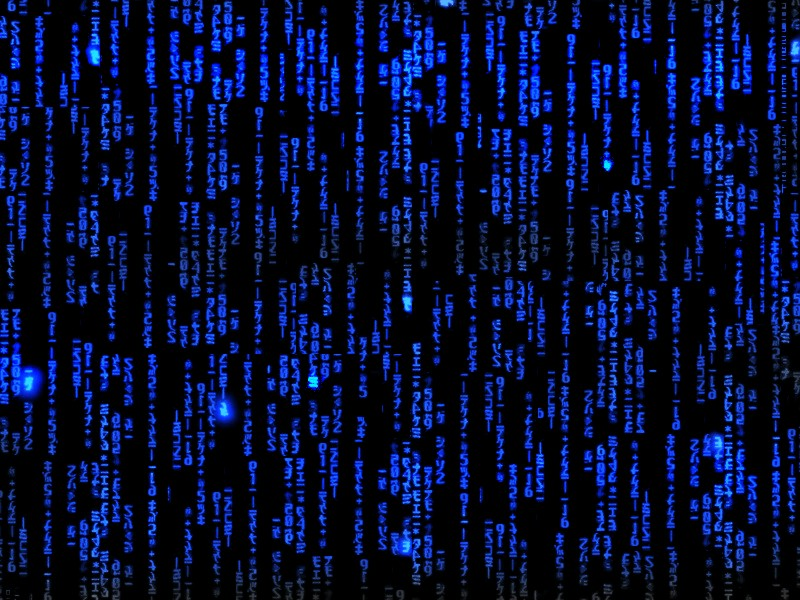Pure Programmer## Project: GCD

The [[Greatest_common_divisor|greatest common divisor]] (GCD) of two integers can be computed with a recursive function by using Euclid's algorithm. Basically if two integers a and b are such that a > b, then the GCD(a, b) is equal to the GCD(a - b, b). Keep reducing the problem through recursion until a = b which is the answer.

Output
\$ g++ -std=c++17 GCD.cpp -o GCD -lfmt \$ ./GCD GCD(6, 9) = 3 GCD(36, 24) = 12 GCD(326, 128) = 2 GCD(49, 121) = 1 GCD(88200, 20790) = 630

Solution